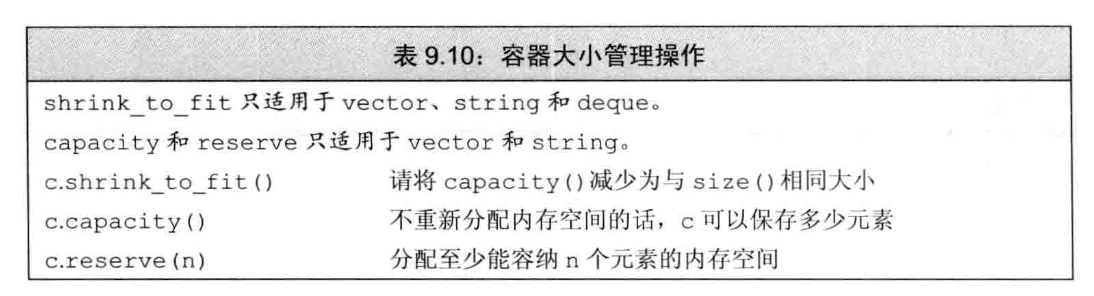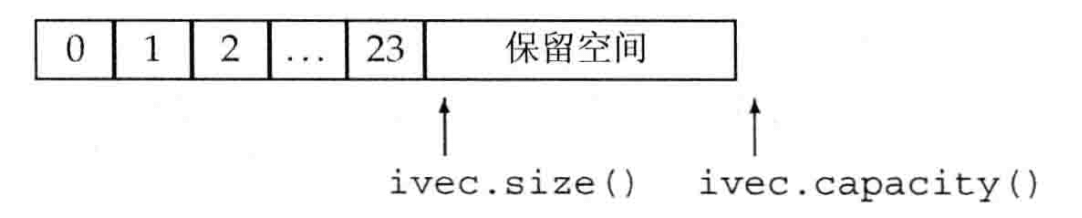# 【C++基础】第五十三课：[顺序容器]vector对象是如何增长的

## shrink_to_fit，capacity，size，reserve

Posted by x-jeff on October 23, 2022

【C++基础】系列博客为参考《C++ Primer中文版（第5版）》C++11标准）一书，自己所做的读书笔记。

# 1.vector对象是如何增长的

## 1.1.管理容量的成员函数reserve并不改变容器中元素的数量，它仅影响vector预先分配多大的内存空间。

## 1.2.capacity和size

1
2
3
4
5
6
7
8
9
vector<int> ivec;
//size应该为0；capacity的值依赖于具体实现
cout << "ivec: size: " << ivec.size() << " capacity: " << ivec.capacity() << endl;
//向ivec添加24个元素
for(vector<int>::size_type ix = 0; ix != 24; ++ix)
ivec.push_back(ix);

//size应该为24；capacity应该大于等于24，具体值依赖于标准库实现
cout << "ivec: size: " << ivec.size() << " capacity: " << ivec.capacity() << endl;


1
2
ivec: size: 0 capacity: 0
ivec: size: 24 capacity: 321
2
3
ivec.reserve(50); //将capacity至少设定为50，可能会更大
//size应该为24；capacity应该大于等于50，具体值依赖于标准库实现
cout << "ivec: size: " << ivec.size() << " capacity: " << ivec.capacity() << endl;


1
ivec: size: 24 capacity: 50


1
2
3
4
5
//添加元素用光多余容量
while (ivec.size() != ivec.capacity())
ivec.push_back(0);
//capacity应该未改变，size和capacity不相等
cout << "ivec: size: " << ivec.size() << " capacity: " << ivec.capacity() << endl;


1
ivec: size: 50 capacity: 50


1
2
3
ivec.push_back(42); //再添加一个元素
//size应该为51；capacity应该大于等于51，具体值依赖于标准库实现
cout << "ivec: size: " << ivec.size() << " capacity: " << ivec.capacity() << endl;


1
ivec: size: 51 capacity: 100


1
2
3
ivec.shrink_to_fit(); //要求归还内存
//size应该未改变；capacity的值依赖于具体实现
cout << "ivec: size: " << ivec.size() << " capacity: " << ivec.capacity() << endl;


1
ivec: size: 51 capacity: 51